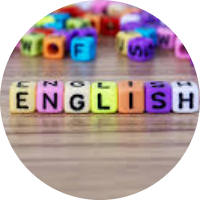# Linear Algebra and its Applications

This set of MCQs helps you brush up on important math topics and prepare you to dive into skill practice.

Start Quiz

The number x = 1.242424.... is expressed in the form x=p/q, where p and q are positive integers having no common factors. Then the value of p−q is,

72

74

76

78

Factorize : 3x−6y+ax−2ay

(x+2y)(3+a)

(x−2y)(3-a)

(xy)(3+a)

(x−2y)(3+a)

Factorize:
4x^2+8x+4

4 (x-3)^2

4 (x+3)^2

4 (x+1)^2

4 (x-1)^2

Write the fraction for the decimal number 0.86 (Reduce your answer to the lowest terms).

43/50

43/25

86/100

23/50

Two cubes have their volumes in the ratio of 1:8. The ratio of their surface areas is,

3:4

2:3

1:4

1:3

What is the distance of point P(6, -6) from the origin?

6

6√2

5√2

7

If the mean of x, x+2, x+4,x+6, x+8 is 11, then the value of x is,

7

3

8

9

###### Evaluate 55×45 using a suitable identity.

2475

2375

2275

2445

Quiz/Test Summary
Title: Linear Algebra and its Applications
Questions: 8
Contributed by: